## Feature scaling and non-negative matrix factorisation

Non-negative matrix factorisation (NMF) is a dimension reduction technique that is commonly applied in a number of different fields, for example:

• in topic modelling, applied to the document x word matrix;
• in speech processing, applied to the matrix of magnitude spectrograms of framed audio;
• in recommendation systems, applied to the user x item interaction matrix.

Due to its non-negativity constraint, it has the wonderful property of decomposing a objects as an additive combination of (often very meaningful) parts. However, as with all unsupervised learning tasks, it is sensitive to the relative scale of different features.

The fundamental problem is that the informativeness of a feature need not be related to its scale. For example, when processing speech, the highest-energy components of a magnitude spectrogram are those of the least perceptual importance! So when NMF decides which information to discard into order to achieve a low-rank factorisation that minimises the error function, it can be the signal, not the noise, that is sacrificed. This problem is not unique to NMF, of course: PCA retains those dimensions of the sample cloud that have the greatest variance.

It is in general better to learn a feature representation jointly with the downstream task, so that the model learns to scale features according to their informativeness for the task. If NMF is for some reason still desirable, however, it is possible to better control the information loss by choosing an appropriate measure of the matrix factorisation error.

There are three common error functions used in NMF (all of which Bregman divergences): squared Euclidean, Kullback-Leibler (KL) and Itakura-Saito (IS). These are respectively quadratic, linear and invariant with respect to the feature scale. Thus, for example, NMF with the Euclidean error function gives strong preference to high-energy features, while NMF with the IS error function is agnostic to feature scale.

## Skipgram isn't Matrix Factorisation

The paper Neural Word Embeddings as Implicit Matrix Factorization of Levy and Goldberg was published in the proceedings of NIPS 2014 (pdf).  It claims to demonstrate that Mikolov’s Skipgram model with negative sampling is implicitly factorising the matrix of pointwise mutual information (PMI) of the word/context pairs, shifted by a global constant.  Although the paper is interesting and worth reading, it greatly overstates what is actually established, which can be summarised as follows:

Suppose that the dimension of the Skipgram word embedding is at least as large as the vocabulary.  Then if the matrices of parametersminimise the Skipgram objective, and the rows ofor the columns ofare linearly independent, then the matrix productis the PMI matrix shifted by a global constant.

This is a really nice result, but it certainly doesn’t show that Skipgram is performing (even implicitly) matrix factorisation.  Rather it shows that the two learning tasks have the same global optimum  – and even this is only shown when the dimension is larger than the vocabulary, which is precisely the case where Skipgram is uninteresting.

### The linear independence assumption

The authors (perhaps unknowingly) implicitly assume that the word vectors on one of the two layers of the Skipgram model are linearly independent.  This is a stronger assumption than what the authors explicitly assume, which is that the dimension of the hidden layer is at least as large as the vocabulary.  It is also not a very natural assumption, since Skipgram is interesting to us precisely because it captures word analogies in word vector arithmetic, which are linear dependencies between the word vectors!  This is not a deal breaker, however, since these linear dependencies are only ever approximate.

In order to see where the assumption arises, first recall some notation of the paper: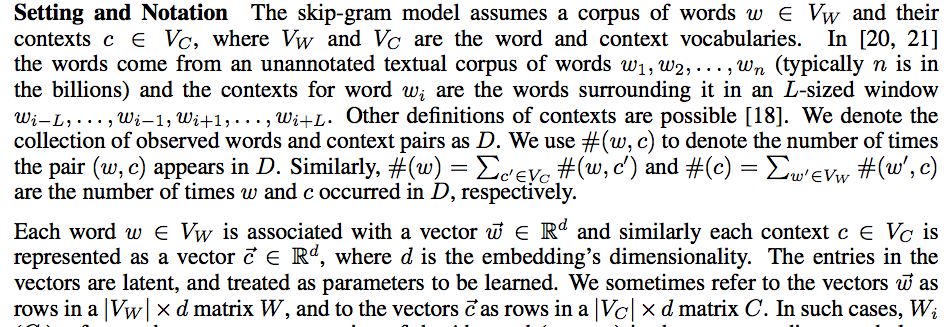The authors consider the case where the negative samples for Skipgram are drawn from the uniform distributionover the contexts, and writefor the log likelihood.  The log likelihood is then rewritten as another double summation, in which each summand (as a function of the model parameters) depends only upon the dot product of one word vector with one context vector: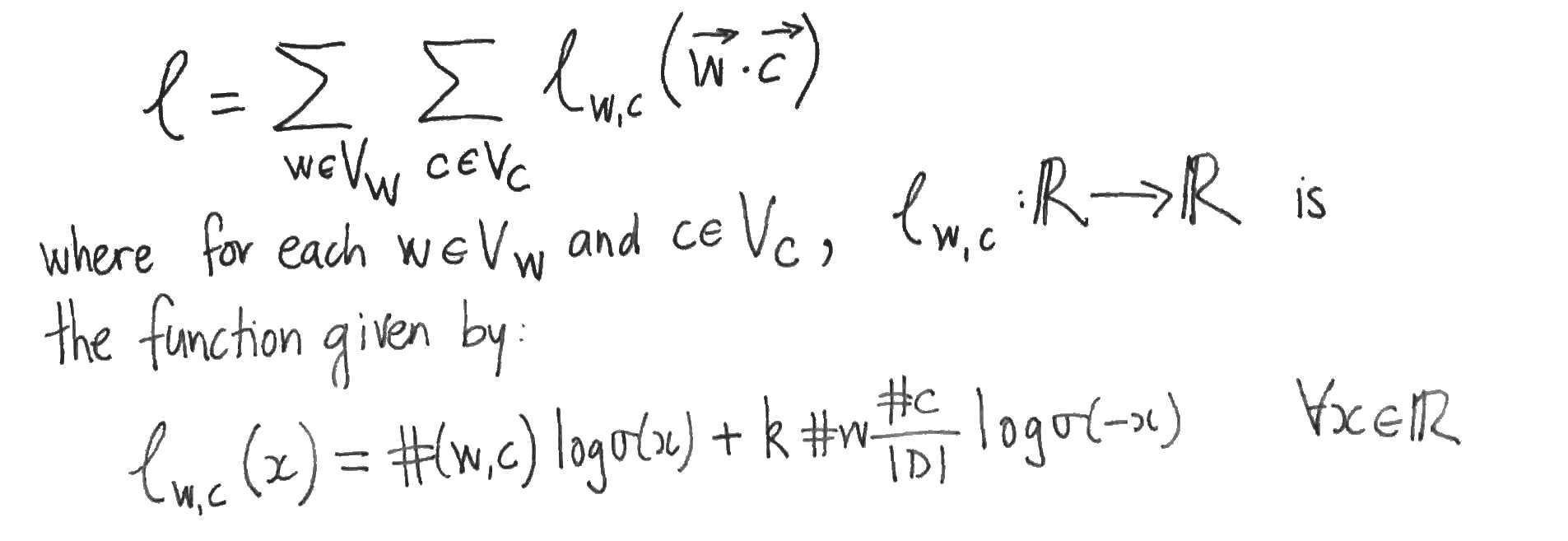The authors then suppose that the values of the parametersare such that Skipgram is at equilibrium, i.e. that the partial derivatives ofwith respect to each word- and content-vector component vanish.  They then assume that this implies that the partial derivatives ofwith respect to the dot products vanish also.  To see that this doesn’t necessarily follow, apply the chain rule to the partial derivatives: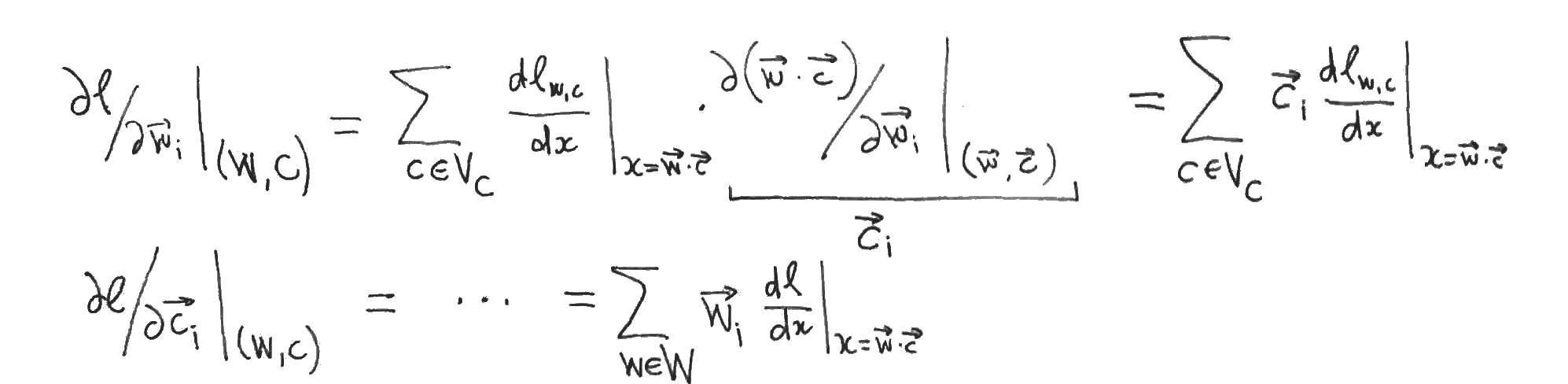This yields systems of linear equations relating the partial derivatives with respect to the word- and content- vector components (which are zero by supposition) to the partial derivatives with respect to the dot products, which we want to show are zero.  But this only follows if one of the two systems of linear equations has a unique solution, which is precisely when its matrix of coefficients (which are just word- or context- vector components) has linearly independent rows or columns.  So either the family of word vectors or the family of context vectors must be linearly independent in order for the authors to proceed to their conclusion.

Word vectors that are of dimension the size of the vocabulary and linearly independent sound to me more akin to a one-hot or bag of words representations than to Skipgram word vectors.

### Skipgram isn’t Matrix Factorisation (yet)

If Skipgram is matrix factorisation, then it isn’t shown in this paper.  What has been shown is that the optima of the two methods coincide when the dimension is larger that the size of the vocabulary. Unfortunately, this tells us nothing about the lower dimensional case where Skipgram is actually interesting.  In the lower dimensional case, the argument of the authors can’t be applied, since it is then impossible for the word- or context- vectors to be linearly independent.  It is only in the lower dimensional case that the Skipgram and Matrix Factorisation are forced to compress the word co-occurrence information and thereby learn anything at all.  This compression is necessarily lossy (since there are insufficient parameters) and there is nothing in the paper to suggest that the two methods will retain the same information (which is what it means to say that the two methods are the same).

### Appendix: Comparing the objectives

To compare Skipgram with negative sampling to MF, we might compare the two objective functions.  Skipgram maximises the log likelihood(above). MF, on the other hand, typically minimises the squared error between the matrix and its reconstruction: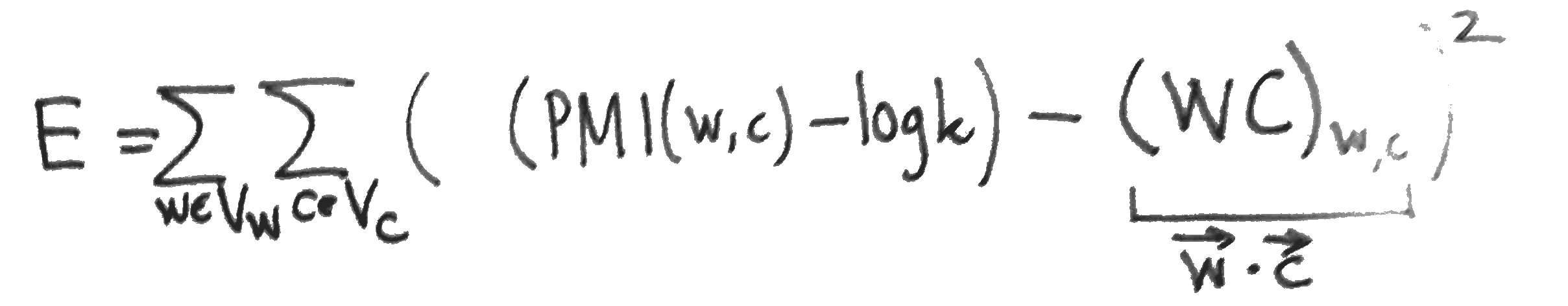The partial derivatives of, needed for a gradient update, are easy to compute: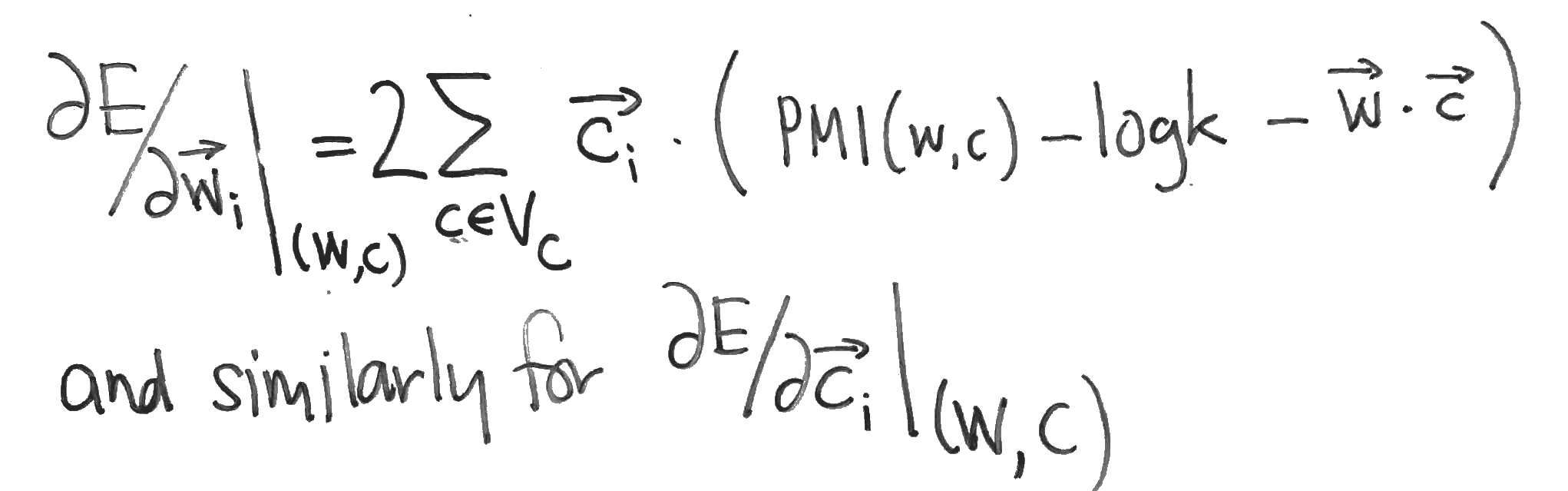Compare these with the partial derivatives of the Skipgram log-likehood, which can be computed as follows: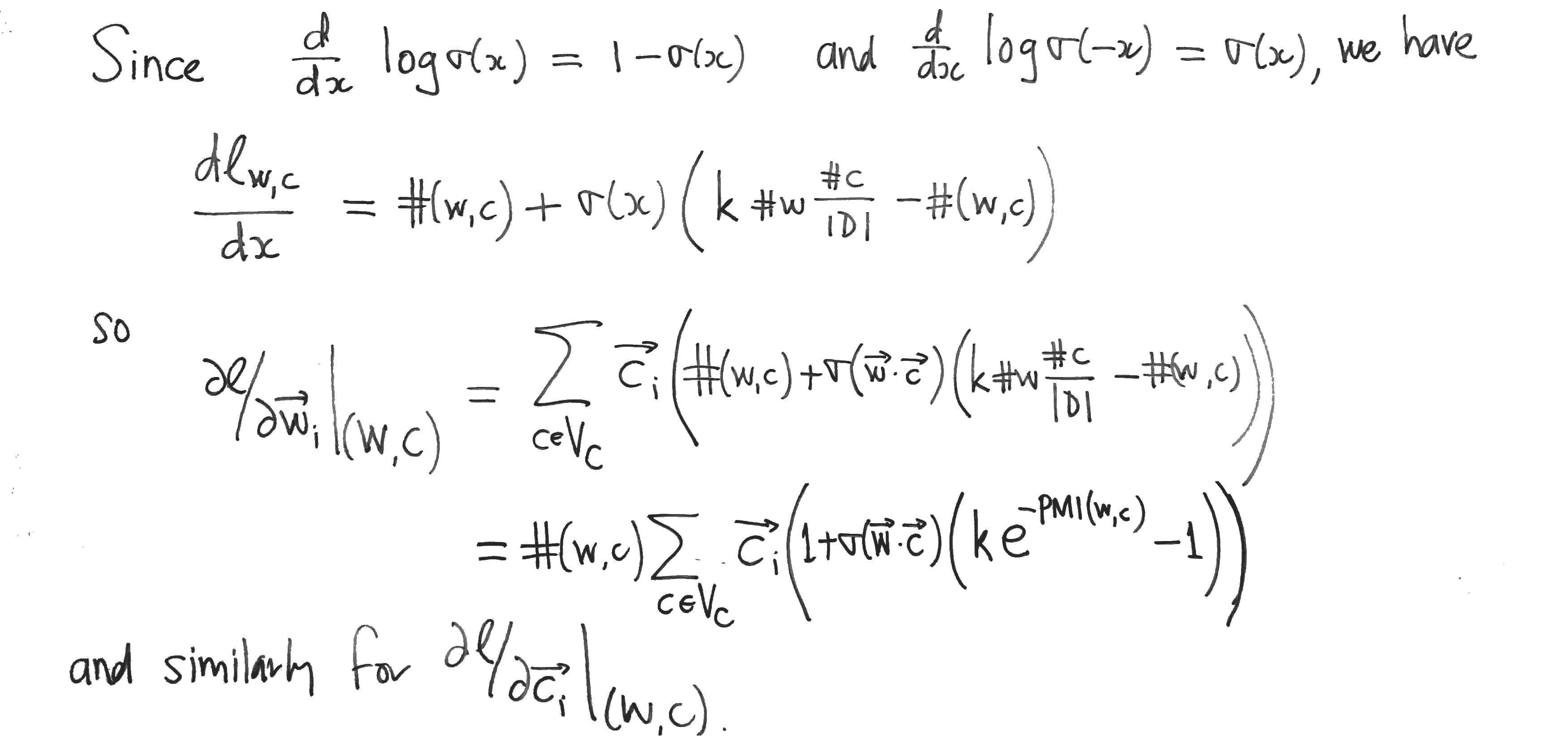## Collaborative Filtering for Implicit Feedback Datasets

Hu, Koren and Volinsky (AT&T, Yahoo!), 2008.

A well-written paper.

PDF

The authors give a good description of the distinctions between explicit and implicit feedback datasets, pointing out in particular that:

1. implicit feedback data is inherently noisy, since a user might decide that they do not like an item after viewing it — interaction does not necessarily indicate interest.
2. the numerical value in explicit feedback indicates preference whereas in the implicit case indicates confidence.

The authors describe their model as being based on SVD, but this is not accurate, since they weight squared difference summands in the cost function according to a confidence value (which is proportional to the number of interactions for that user-item pair).

The input matrix is the user-item matrix.

Optimisation is via alternating least squares.

Their evaluation metric is percentile rank based.

Their model, which we’ll call “weighted SVD” (they speak of “confidence intervals”) compares favourably with the baseline popularity method and also with an old-school item-based neighbourhood method, in terms of the expected percentile rank (Figure 1). Interestingly, the differences are less marked when the probability that a desired item is in the top (say) 1% is considered (Figure 2).

The unweighted SVD on the user-item matrix is shown to perform terribly, with a significant but insufficient improvement obtained with regularisation.

## Matrix Factorisation and the Eigendecomposition of the Gram Matrix

Here we consider the problem of approximately factorising a matrixwithout constraints and show that solutions can be generated from the orthonormal eigenvectors of the Gram matrix(i.e. of the sample covariance matrix).

For this we need the eigendecomposition of real symmetric matrices.

Questions, all related to one another:

• What other solutions are there?
• (Speculative) can we characterise the solutions as orbits of the orthogonal group on the solutions above, and on those solutions obtained from the above by adding rows of zeros to?
• Under what constraints, if any, are the optimal solutions to matrix factorisation matrices with orthonormal rows/columns? To what extent does orthogonality come for free?